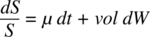# Documentation/Calc Functions/OPT PROB INMONEYUnder construction. For now, see the system Help files.

OPT_PROB_INMONEY

## Category:

Financial Analysis

## Summary:

Returns the probability that an asset will end up between two barrier levels at maturity, assuming that the stock price can be modeled as a process S that follows the stochastic differential equation, as follows.µ is the asset’s percentage drift, vol is the percentage volatility of the stock, and dW is a random sample drawn from a normal distribution with a zero mean. W is a Wiener process or Brownian motion.

## Syntax:

OPT_PROB_INMONEY(Spot; Volatility; Drift; Maturity; Lower Barrier; Upper Barrier; Strike; Put Call)

## Arguments:

Spot is the price / value of the underlying asset and should be greater than 0.0.

Volatility is the annual percentage volatility of the underlying asset expressed as a decimal (for example, enter 30% as 0.3). The value should be greater than 0.0.

Drift is the annual stock price percentage drift rate (µ in the above formula). The value is expressed as a decimal (for example, enter 15% as 0.15).

Maturity is the time to maturity of the option, in years, and should be non-negative.

Strike is the strike price of the option and should be non-negative.

Put Call is a string that defines whether the option is a put (“p”) or a call (“c”).

If the optional Strike and Put Call arguments are included, then

• For a call option, the function returns the probability that the asset will end up between Strike and Upper Barrier.
• For a put option, the function returns the probability that the asset will end up between Lower Barrier and Strike.

The function ignores the possibility of knock-out before maturity.

For relevant background information, visit the Options (finance) and Black-Scholes model Wikipedia pages.

## Examples:

=OPT_PROB_INMONEY(30;0.2;0.1;1;0;50) returns the value 0.9844.

=OPT_PROB_INMONEY(70;0.3;0.15;1;60;0;80;"p") returns the value 0.3440.